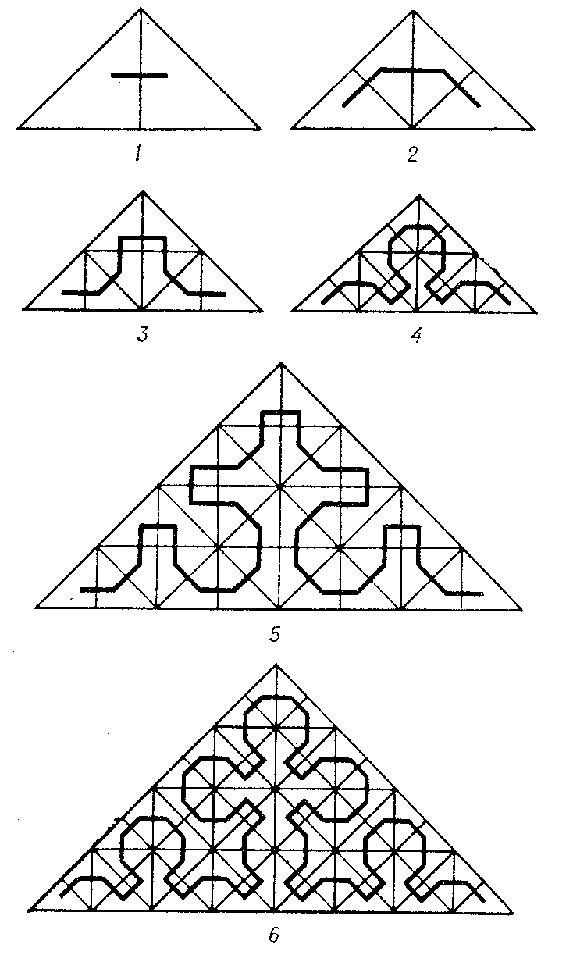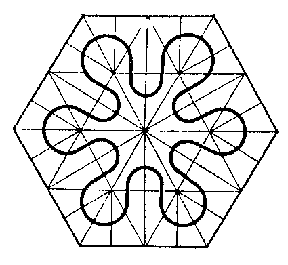# Peano curve

A continuous image of a segment filling the interior of a square (or triangle). It was discovered by G. Peano .Figure: p071890a

A Peano curve, considered as a plane figure, is not a nowhere-dense plane set; it is a curve in the sense of Jordan, but not a Cantor curve, therefore it does not have a length. For a construction of a Peano curve filling a square, due to D. Hilbert, see Line (curve). In Fig. aan analogue of his construction for a triangle (the first six steps) has been drawn (for other constructions, see  and ).

Every Peano curve has multiple points. "This proposition is of enormous importance in geometry, for it shows precisely the geometric essence of the difference of the dimensions of the plane and the line" (N.N. Luzin). There are no Peano curves at which every point is simple or two-fold, but there is a Peano curve with as multiple points (only countably many) three-fold ones. Such is, for example, the one constructed by Peano himself; Hilbert's construction contains (also, countably many) four-fold points.

Connected with the notion of a Peano curve is the curious fact that there exist simple arcs in space that can be projected into the plane in the form of solid areas — such is, for example, a curve $x=\phi(t)$, $y=\psi(t)$, $z=t$, where the first two functions give a Peano curve. Although this arc is impermeable to rain, it is by no means a continuous surface.

There is a certain interest in so-called regular closed curves of Peano type — limits of sequences of symmetric closed curves corresponding to sequences of triangulations of an arbitrary regular polygon, each of which is a regular (that is, obtained by division into two equal parts) subdivision of the preceding one (for an example, see Fig. b). The sequence of curves can be chosen so that the limit of the areas of the domains bounded by them is equal to a given quantity (even zero or the arc of the whole figure to be subdivided (Fig. c)). It seems probable that similar figures can be useful in studying the growth of crystallic structures. Similarly, by means of sequences of triangulations one can construct mappings of a line into a plane, in particular, "periodic" curves of Peano type.Figure: p071890bFigure: p071890c

There is an analogue of a Peano curve filling a multi-dimensional, and even a countably-dimensional, cube (see ).

A far-reaching generalization is the theorem of Mazurkiewicz: If $X$ is a continuum, then the conditions a) $X$ is a locally connected space and b) $X$ is the continuous image of an interval, are equivalent.

How to Cite This Entry:
Peano curve. Encyclopedia of Mathematics. URL: http://encyclopediaofmath.org/index.php?title=Peano_curve&oldid=32355
This article was adapted from an original article by M.I. Voitsekhovskii (originator), which appeared in Encyclopedia of Mathematics - ISBN 1402006098. See original article